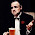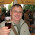Monday, 11 April 2011

Calculating the pH of old beers

Looking at the figures for historical beers that sometimes appear in some of my favourite blogs I've found it frustrating that acidity is given as a decimal figure, not as the pH that I'm familiar with. The pH scale was developed in 1909 by Sørensen at the Carlsberg brewery laboratory, so was first used in research on beer, but compared to how long beer has been around it is still relatively recent which I guess is why it's not used.

As the beer historian bloggers aren't scientists they've been unable to help me to convert acidity to pH. But as I am a scientist I thought I'd give it a go at working it out myself. It's been a while since I've done calculations like this but it's good to try knocking the rust off my drink addled brain every now and then.

If calculations about acidity in historic beer don't interest you look away now.

From a recent post at Ron's Shut up about Barclay Perkins we get an analysis of Dreher's Vienna lager that lists acetic acid as 0.12.

This I take to be a percentage volume, so I'll divide it by 100 to get the concentration [0.0012].

The disassociation constant (Ka) of acetic acid is 1.75 x 10e-5 and its chemical formula is CH3COOH.

As acetic acid is a weak acid I'm using the simplified formula:

Ka = [H+][CH3COO-]/[CH3COOH]

(so to put in the figures we have gives us)

1.75 x 10e-5 = [H+][CH3COO-]/0.0012

(multiply this by 0.0012 to get)

2.1 x 10e-8 = [H+][CH3COO-]

(as [H+]= [CH3COO-] we have)

2.1 x 10e-8 = [H+] squared

(take the square root)

1.45 x 10e-4 = [H+]

(as pH = -log[H+] )

(find the log)

(take away the minus sign)

Which gives us the pH as 3.83

When we made lager during my studies at Heriot-Watt the final pH was 3.84 so this looks like a good figure for a lager pH to me.

For comparison Murphy and Son give an acceptable pH range for cask conditioned ales of 3.7 to 4.1 and a pH of 3.3 for lambic beer is given here.

Looking at some of Ron's other figures we can see acidities for a range of barley wines. Applying the same formula to the most acidic (1870 Bass barley wine at 0.23 acid) we get a pH of 3.69 and for the least acidic (various at 0.04 acid) we get pH 4.07, so I'm feeling fairly confident in my workings and my assumptions that brewing chemists' acidity scale was based on acetic acid and expressed as a percentage. Though if anyone knows better or spots an error I've made I'm all ears!

This free acid is represented in the tables as acetic acid; but there is reason to believe that only a part of it is acetic acid, and that beer probably contains lactic acid and some substance analogous to glucic acid, which, according to Graham, Hofmann, and Redwood, appears to be produced in the fermentation of beer-worts, as practised in this country.

1.Can you also explain the 19th century method of declaring the amount of CO2 in beer? That makes my head hurt.

2.I'm a bit worried my brain will overheat as it is but if you can give me a link to some figures I'll look into it.

3.I get pH 3.84 which is close enough for government work.

The figures Ron has posted today showing acidity of 0.13, 0.05, and 0.06 give pH values of 3.82, 4.03, and 3.99 all of which seem reasonable so I guess you are right. Hooray!

4.FWIW I use this spreadsheet formula:

=LOG(SQRT(B5*A5/100))*-1

where B5 is the dissociation constant expressed as a decimal and A5 the acidity value as a percent (the figure published in other words).

5.Nice one mentaldental. After I'd worked out the figures using a calculator and notepad (which is no doubt where the rounding error crept in) I did make an excel file, but it lacked the elegance of your formula as it was spread across six columns!